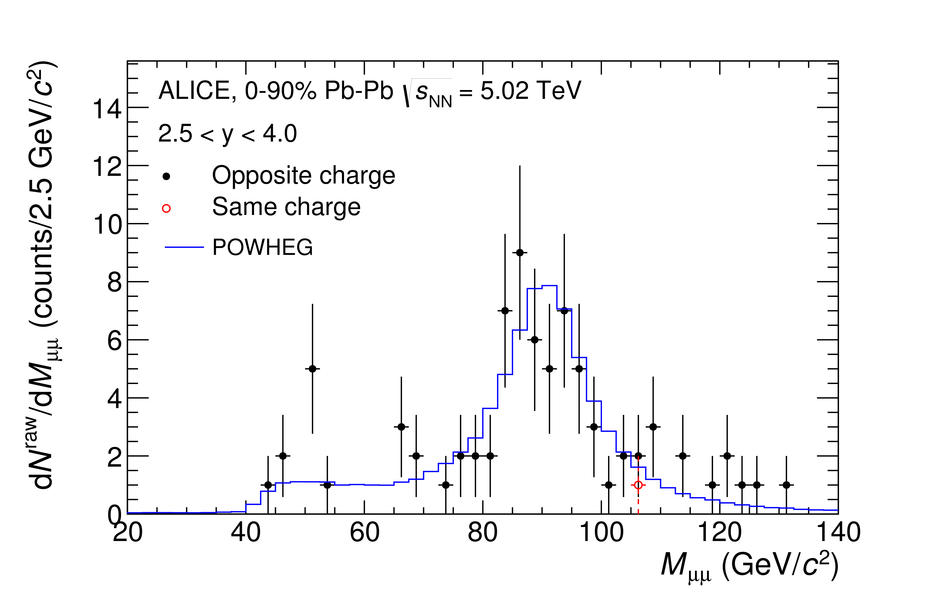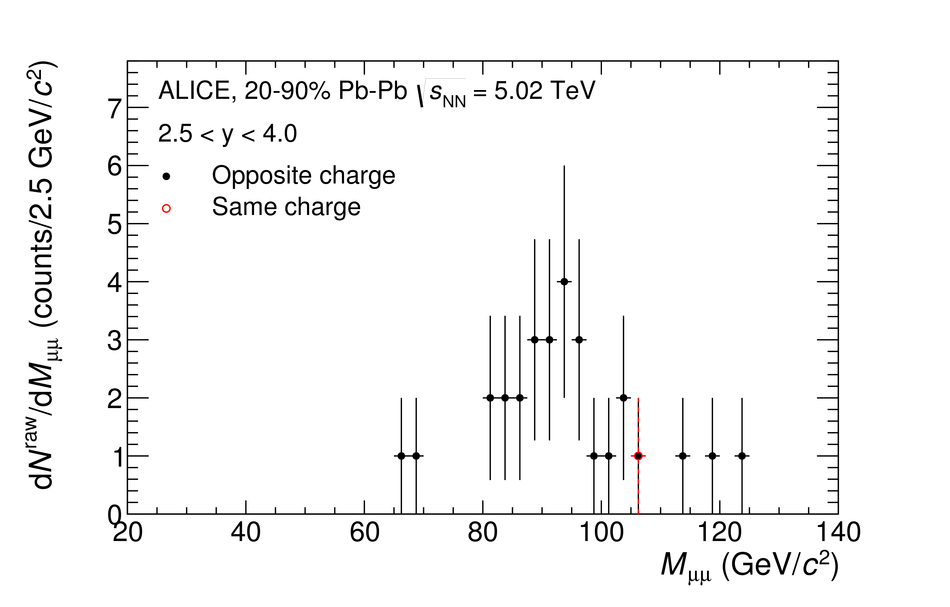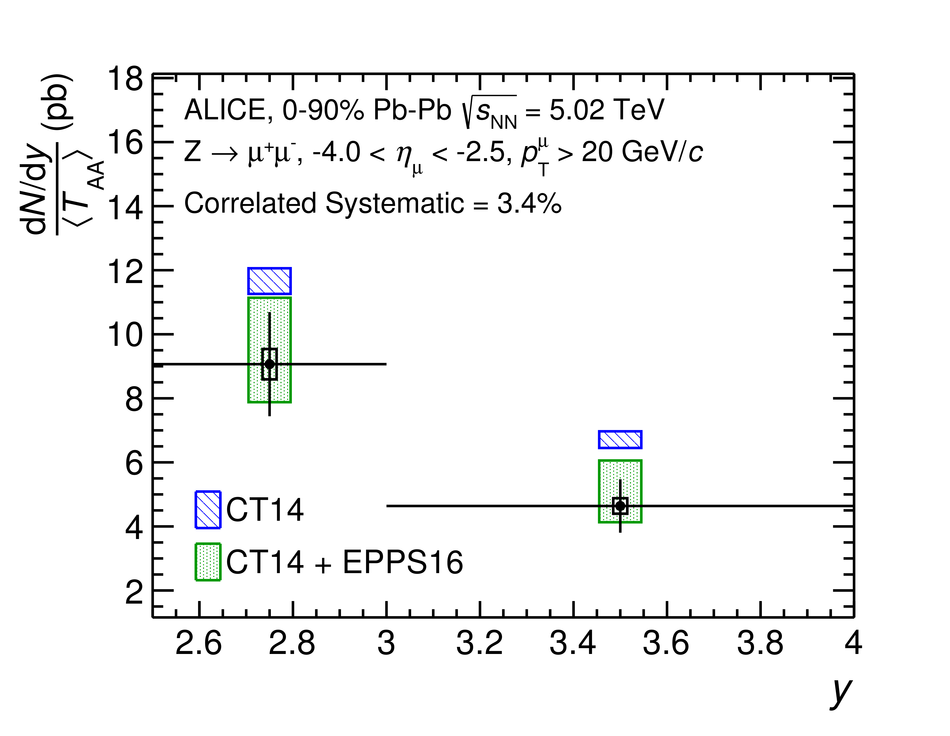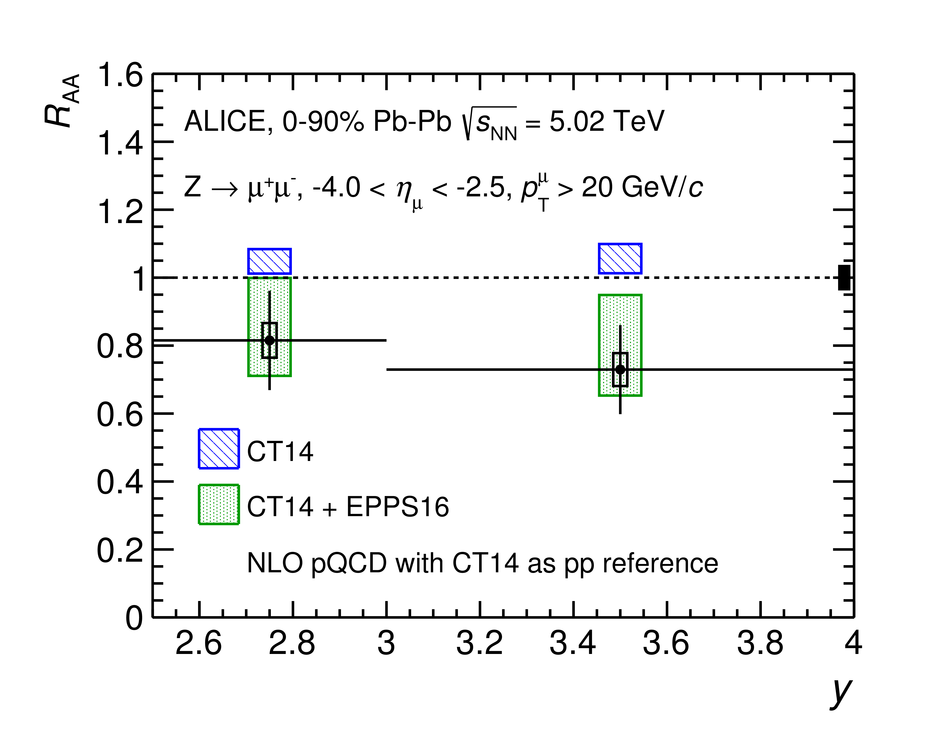Measurement of Z$^0$-boson production at large rapidities in Pb-Pb collisions at $\sqrt{s_{\rm NN}}=5.02$ TeV

The production of Z$^0$ bosons at large rapidities in Pb-Pb collisions at $\sqrt{s_{\rm NN}}=5.02$ TeV is reported. Z$^0$ candidates are reconstructed in the dimuon decay channel (${\rm Z}^0 \rightarrow \mu^+\mu^-$), based on muons selected with pseudo-rapidity $-4.0<~\eta<~-2.5$ and $p_{\rm T}>20$ GeV/$c$. The invariant yield and the nuclear modification factor, $R_{\rm AA}$, are presented as a function of rapidity and collision centrality. The value of $R_{\rm AA}$ for the 0-20% central Pb-Pb collisions is $0.67 \pm 0.11 \, \mbox{(stat.)} \, \pm 0.03 \, \mbox{(syst.)} \, \pm 0.06 \, \mbox{(corr. syst.)}$, exhibiting a deviation of $2.6 \sigma$ from unity. The results are well-described by calculations that include nuclear modifications of the parton distribution functions, while the predictions using vacuum PDFs deviate from data by $2.3\sigma$ in the 0-90% centrality class and by $3\sigma$ in the 0-20% central collisions.

Figures

Figure 1

 Invariant mass distribution of \dimuon pairs for \PbPb collisions at $\snn=5.02$~TeV, reconstructed using muons with $-4.0< \etamu< -2.5$ and $\ptmu>20$\GeVc (black points) The panels present the distribution in different centrality intervals. The error bars are of statistical origin only The invariant mass distribution of like-sign muon pairs is also shown (red open points). Only one like-sign candidate is found in the \centr{20}{90} centrality interval The solid blue line drawn in \fig{fig:ZRawYield_0_90} represents the distribution from a POWHEG simulation for \PbPb collisions without nuclear modification of PDFs (see text for details)Figure 2

 Invariant yield of \dimuon from \zBoson production in $2.5< y< 4.0$ divided by the average nuclear overlap function in the \centr{0}{90} centrality class, considering muons with $-4.0< \etamu< -2.5$ and $\ptmu>20$\GeVc. The horizontal solid line represents the statistical uncertainty of the measurement while the yellow filled band shows the systematic uncertainty. The result is compared to theoretical calculations with and without nuclear modification of the PDFs~ All model calculations incorporate PDFs or nPDFs determined by considering the isospin of the Pb-nucleusFigure 3

 Invariant yield of \dimuon from \zBoson in $2.5< y< 4.0$ divided by \taa (a) and nuclear modification factor (b) as a function of rapidity for \PbPb collisions at $\snn=5.02$~TeV, considering muons with $-4.0< \etamu< -2.5$ and $\ptmu>20$\GeVc The vertical error bars are statistical only. The horizontal error bars display the measurement bin width, while the boxes represent the systematic uncertainties The filled black box in panel (b), located at $\Raa=1$, shows the normalisation uncertainty The results are compared to theoretical calculations with and without nuclear modification of the PDFs The filled blue boxes show the calculation using the CT14 PDF, while the green stippled boxes show the calculation using CT14 PDF with EPPS16 nPDF~ All model calculations incorporate PDFs or nPDFs that account for the isospin of the Pb nucleusFigure 4

 Invariant yield of \dimuon from \zBoson in $2.5< y< 4.0$ divided by \taa (a) and nuclear modification factor (b) as a function of centrality (represented by \npartNcoll) for \PbPb collisions at $\snn=5.02$~TeV, considering muons with $-4.0< \etamu< -2.5$ and $\ptmu>20$\GeVc The vertical error bars are statistical only, while the boxes represent the systematic uncertainties The filled black box in panel (b), located at $\Raa=1$, shows the normalisation uncertainty The results are compared to theoretical calculations with centrality-dependent nPDFs that account for the isospin of the Pb nucleus~